# Introduction

This vignette is devoted to show the application of iccCounts package to assess the repeatability and concordance with some examples where the outcomes are counts. In both analyses (repeatability and concordance), repeated measures are taken on a sample of subjects and the agreement among the repeated measures from the same subjects is assessed. In the repeatability analysis the repeated measures are interchangeable (replicates) whilst in the concordance analysis they are structured (not interchangeable) because they were obtained by different methods.

The index used to assess the agreement is the intraclass correlation coefficient (ICC) (Fleiss, 1986) which in the case of the concordance analysis is equivalent to the concordance correlation coefficient (Lin, 1989; Carrasco and Jover, 2003).

In the case of count data, the ICC is estimated by means a generalized linear mixed model (GLMM) with a subject’s (cluster) random effect distributed as a Normal distribution with 0 mean and variance $$\sigma_b^2$$. The expression of the ICC depends on the assumed within-subjects distribution (Carrasco, 2010). The GLMM is estimated using the glmmTMB package (Brooks et al., 2017).

As a first analysis, the Poisson distribution will be considered. However, the validity of the ICC estimate is closely linked to the validity of the model. Thus, a goodness-of-fit (GOF) analysis of the model has to be carried out. The GOF analysis will involve the computation of randomized quantile residuals (RQR) (Dunn, 1996; Feng et al. 2020). The RQR from the original data will be compared to those obtained by simulation under the fitted model. The number of simulation by default is 100, but it can be increased to get more precision. Thus, simulated data are generated using the assumed model and their sample estimates. The model is refitted at each simulation and the RQR computed.

Using the original and simulated RQR the GOF will involve:

• Envelopes. Computing envelopes as the minimum and maximum RQR of each measure from the simulated samples. In case the model fits appropriately the data all the original RQR should lie within the envelopes.

• Dispersion. The variance of the RQR will be computed and compared to those from the original samples. The proportion of simulated variances that are greater than that from the original sample will be used as a simulated P-value to test whether the data dispersion is well fitted by the model. In case of rejecting this hypothesis, models that can afford larger dispersion should be accounted for.

• Zero inflation. One issue that can arise with count data is the zero inflation, i.e. more zeros in the sample than expected by the assumed model. To check this question, the proportion of zeros in the original sample will be compared to those from the simulated samples. The proportion of zeros in the simulated samples that are greater than that from the original sample will be used as a simulated P-value to test whether the proportion of zeros is well fitted by the model. In case of rejecting this hypothesis, models that include zero inflation will be applied.

# Example 1. CD34+ count cell data

A new method of flow cytometry for counting CD34+ cells is compared to the readings obtained by the standard approach (Fornas et al., 2000). Both were applied to a sample of 20 subjects. In the dataset, the new and standard methods are coded as 1 and 3 respectively.

library(iccCounts)

Let’s estimate the ICC assuming the within-subjects pdf is Poisson. The function icc_counts is a wrapper that will execute the analysis. The function will give as a result an object of class iccc that is a list with the following components:

• model. The GLMM estimated using the glmmTMB package. It is a glmmTMB object, so that all the features related to glmmTMB objects can be applied on it.

• ICC. Estimate of the ICC, its standard error and confidence interval. The iccCounts package also includes the ICC function that applied to the iccc object will print this component.

• varcomp. Variance components and parameters related to ICC expression. The iccCounts package also includes the VarComp function that applied to the iccc object will print this component.

Additionally, because we are facing a concordance analysis, the name of the method variable has to be provided using the met argument along specifying the type of analysis in the type argument.

AF_P<-icc_counts(AF,y="y",id="id",met="met",type="con")

The estimate of the ICC is:

ICC(AF_P)
#> Model: poisson
#>            ICC   SE ICC 95% CI LL 95% CI UL
#> [1,] 0.8472696 0.021989 0.7982025 0.8851678

and the variance components estimates are:

VarComp(AF_P)
#> Model: poisson
#>       mu    BSVar     BMVar
#>  761.809 1.234619 0.1199439

where mu stands for the overall expectation, BSVar is the variance of the subject’s random effect, and BMVar expresses the between-methods variability.

Nevertheless, as it was said before, the validity of the estimates is related to the validity of the model. To check that the function GOF_check is applied on the iccc object. The execution takes some time (46 seconds in i7-CPU at 1.99GHz with 16Gb of RAM). The random seed is fixed to make the result reproducible though differences between simulations should be small with moderate to large number of simulations.

set.seed(100)
#AF_P.gof<-GOF_check(AF_P)

First, let’s draw the plot of RQR envelopes.

plot(AF_P.gof,type="envelope")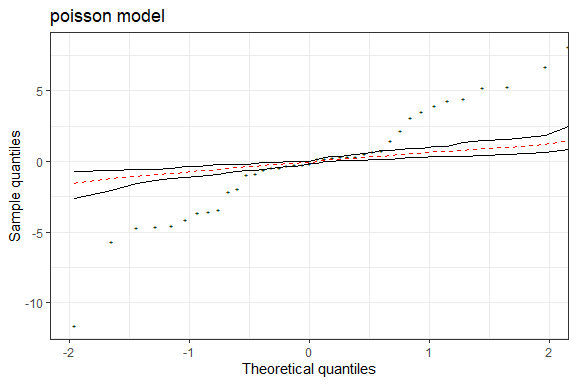Several points lie outside of envelopes so the model does not fit appropriately the data.

Let’s see if the problem is on the dispersion and/or the number of zeros.

plot(AF_P.gof,type="dispersion")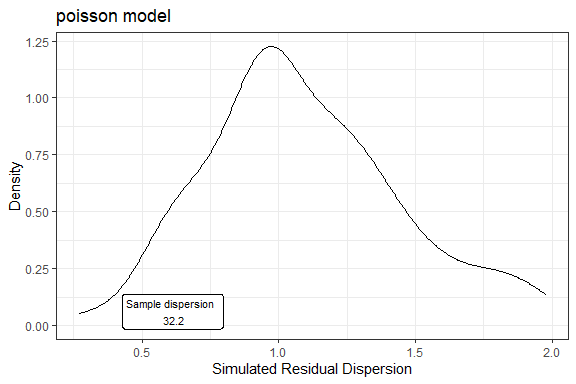DispersionTest(AF_P.gof)
#>         S    P_value
#>  32.20049 0.00990099

The dispersion of the RQR from the original sample is very much greater than that from the simulated RQR. So that, the model assuming a Poisson pdf do not fit well the actual dispersion of data.

On the other hand, there is no problem with the number of zeros.

plot(AF_P.gof,type="zeros")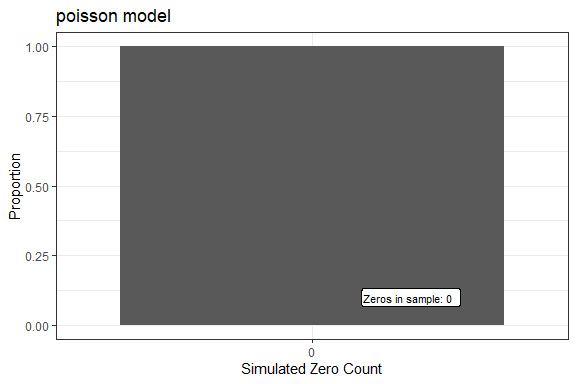ZeroTest(AF_P.gof)
#>  Count P_value
#>      0       1

Let’s try to fit the model with Negative Binomial pdf to afford a larger dispersion. The family “nbinom1” involves an additive extradispersion, $$Var\left(y_i\right)=\mu_i\left(1+r\right)$$, while “nbinom2” family considers a proportional extradispersion, $$Var\left(y_i\right)=\mu_i\left(1+r\mu_i\right)$$. To check which model fits better the data the generic function AIC is applied to the glmmTMB object.

AF_NB1<-icc_counts(AF,y="y",id="id",met="met",type="con", fam="nbinom1")
AF_NB2<-icc_counts(AF,y="y",id="id",met="met",type="con", fam="nbinom2")
AIC(AF_NB1$model) #>  579.0913 AIC(AF_NB2$model)
#>  576.9105

The Negative Binomial with proportional extradispersion has a slightly lower AIC. Let’s check the goodness of for for this model.

set.seed(100)
#AF_NB2.gof<-GOF_check(AF_NB2)

In the The plot of RQR envelopes, all the original RQR lie within the envelopes, so the model fits correctly the data.

plot(AF_NB2.gof,type="envelope")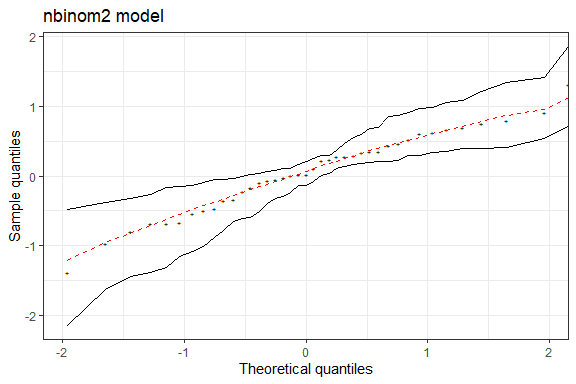The dispersion of the original RQR is compatible with those simulated RQR, so that the dispersion estimated by the model is now correct.

plot(AF_NB2.gof,type="dispersion")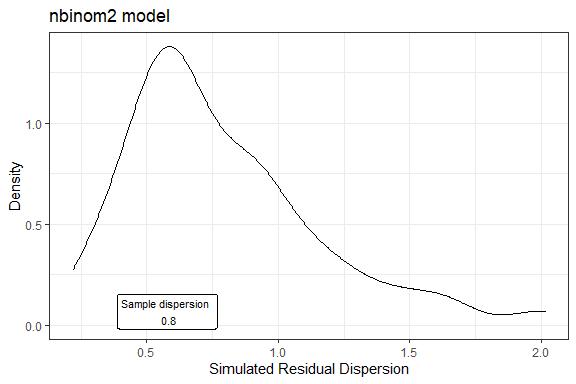DispersionTest(AF_NB2.gof)
#>          S   P_value
#>  0.8002765 0.4059406

Finally, the ICC estimate using this is:

ICC(AF_NB2)
#> Model: nbinom2
#>           ICC    SE ICC 95% CI LL 95% CI UL
#> [1,] 0.834794 0.0454062 0.7212048 0.9046669

and the variance components

VarComp(AF_NB2)
#> Model: nbinom2
#>        mu    BSVar     BMVar         r
#>  777.1946 1.188904 0.0809433 0.0488122

# Example 2. Tick counts data

In this study, the repeatability of line transects survey method to estimate tick abundance was assessed (Kjellander et al., 2021). With this aim, sampling was performed by two parallel transects separated by 1m-2m where the total count of ticks was recorded. In this analysis, every pair of transects are considered as replicates of a common transect.

The ICC estimate assuming a Poisson distribution for the within-subjects variability is:

G_P<-icc_counts(Grimso,y="Tot",id="TransectID")
ICC(G_P)
#> Model: poisson
#>            ICC    SE ICC 95% CI LL 95% CI UL
#> [1,] 0.3494333 0.1369518 0.0589753 0.5853431
VarComp(G_P)
#> Model: poisson
#>         mu    BSVar
#>  0.2072297 1.278685

When checking the GOF, if the plot function is applied with no value in the type argument, the three plots (envelopes, dispersion and zeros) are drawn.

set.seed(100)
#G_P.gof<-GOF_check(G_P)
plot(G_P.gof)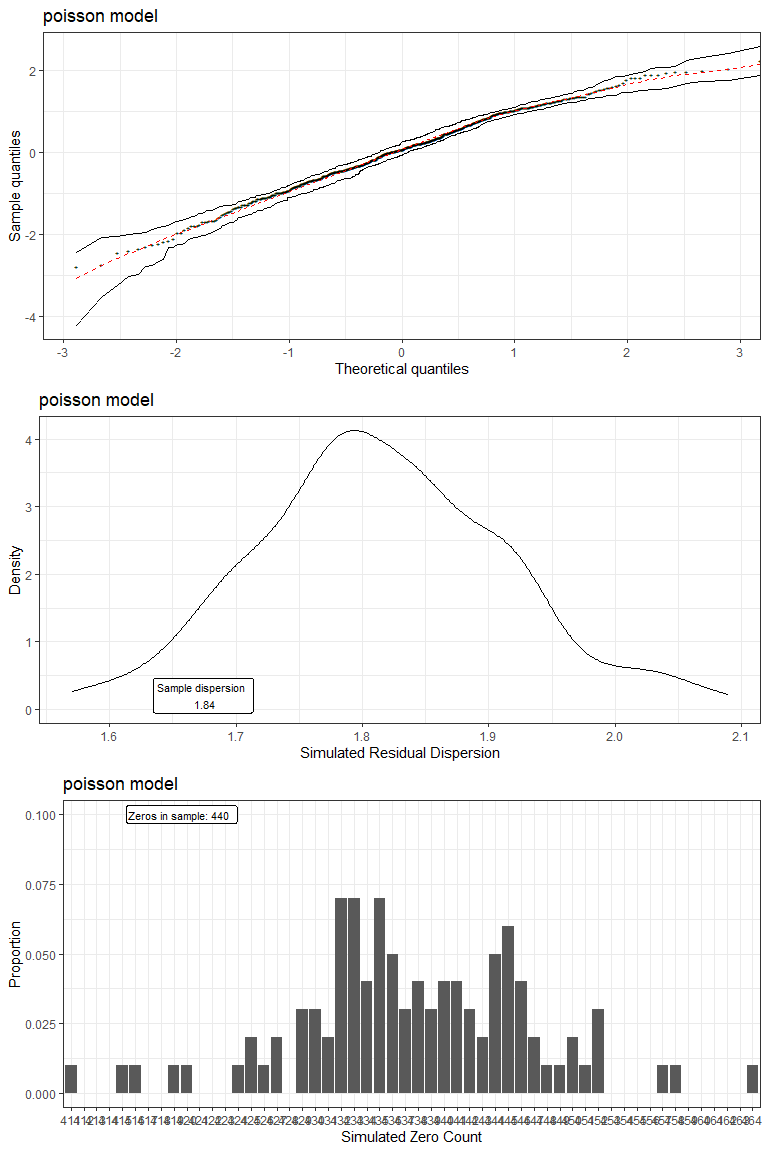All the RQR are within the envelopes. Furthermore, the dispersion and the zero count are well fitted by the model.

DispersionTest(G_P.gof)
#>        S   P_value
#>  1.83724 0.4158416
ZeroTest(G_P.gof)
#>  Count   P_value
#>    440 0.4158416

# Example 3. Sparrow fledglings paternity

The incidence of extra-pair paternity (EPP) was monitored over 3 breeding seasons in a sparrow colony in Lundy, an island off the southwest coast of England (Schroeder et al., 2012). Here, the repetability of counts of fledglings a male had in every breeding season is assessed.

Let’s begin by estimating the ICC assuming a Poisson distribution for the within-subjects variability,

EPP_P<-icc_counts(EPP,y="Social",id="id")
ICC(EPP_P)
#> Model: poisson
#>            ICC    SE ICC 95% CI LL 95% CI UL
#> [1,] 0.6234229 0.0886235 0.4189835 0.7677034
VarComp(EPP_P)
#> Model: poisson
#>        mu     BSVar
#>  3.278005 0.4088144

Next, let’s check the GOF.

set.seed(100)
#EPP_P.gof<-GOF_check(EPP_P)
plot(EPP_P.gof,type="envelope")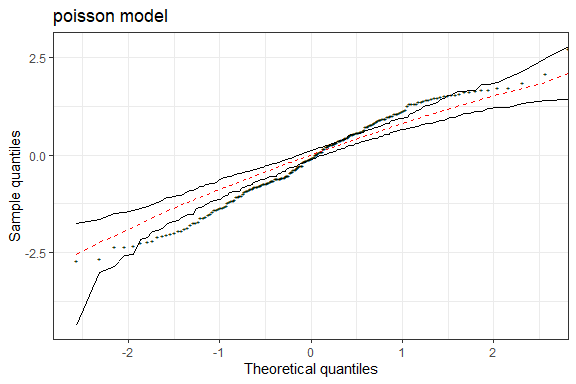The envelopes plot show some residuals that lie outside the envelopes.

plot(EPP_P.gof,type="dispersion")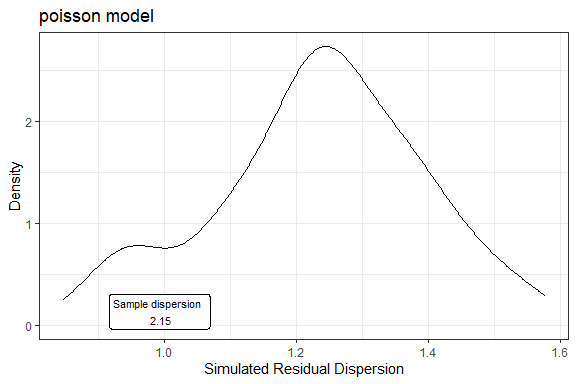DispersionTest(EPP_P.gof)
#>         S    P_value
#>  2.154477 0.00990099

The dispersion is also greater than expected under a Poisson model. Finally, with regard of zero counts,

plot(EPP_P.gof,type="zeros")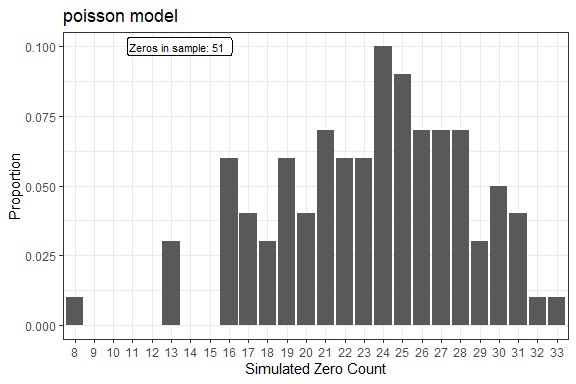ZeroTest(EPP_P.gof)
#>  Count    P_value
#>     51 0.00990099

The number of zeros in the sample is larger than expected under the Poisson assumption.

Thus, it is necessary to try different models that can afford larger dispersion and number of zeros. Let’s check if the negative binomial model can be such a model.

EPP_NB1<-icc_counts(EPP,y="Social",id="id",fam="nbinom1")
EPP_NB2<-icc_counts(EPP,y="Social",id="id",fam="nbinom2")
AIC(EPP_NB1$model) #>  887.0502 AIC(EPP_NB2$model)
#>  901.182

In this case, the negative binomial with additive extradispersion fits the data better. Let’s check the GOF for this model.

set.seed(100)
#EPP_NB1.gof<-GOF_check(EPP_NB1)
plot(EPP_NB1.gof,type="envelope")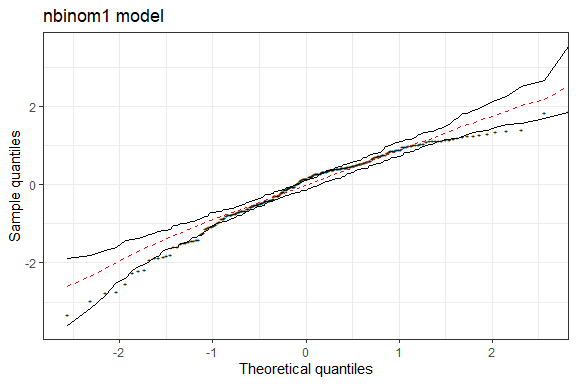In the envelopes plot, the RQR behave much better than in the Poisson case, However, there still are some points that lie outside the envelopes.

plot(EPP_NB1.gof,type="dispersion")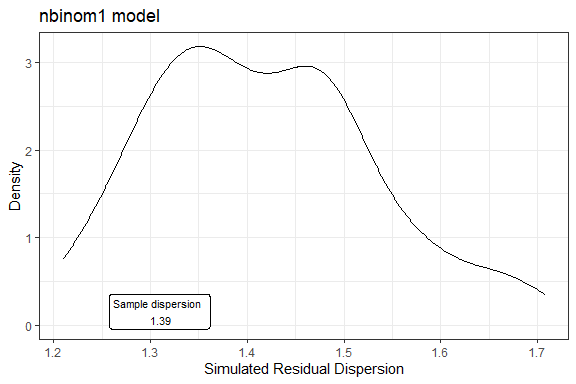DispersionTest(EPP_NB1.gof)
#>        S   P_value
#>  1.39092 0.5346535

On the other hand, the sample dispersion is compatible to that from the simulated samples.

plot(EPP_NB1.gof,type="zeros")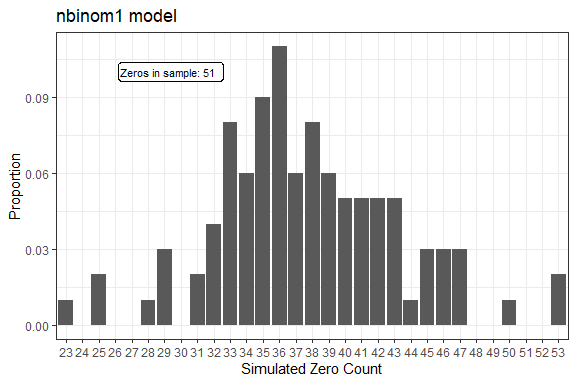ZeroTest(EPP_NB1.gof)
#>  Count    P_value
#>     51 0.02970297

Finally, there still is an excess of zero counts. Hence, it is necessary to apply a model able to account for a larger number of zeros.

Let’s try with the zero inflated Poisson model (ZIP). The ICC and variance components for this model are:

EPP_ZIP<-icc_counts(EPP,y="Social",id="id",fam="zip")
ICC(EPP_ZIP)
#> Model: poisson zero inflated
#>            ICC    SE ICC  95% CI LL 95% CI UL
#> [1,] 0.0445696 0.0347733 -0.0236865 0.1124121
VarComp(EPP_ZIP)
#> Model: poisson zero inflated
#>        mu     BSVar        Pi
#>  4.373881 0.0299266 0.2513053

Notice the excess of zeros is about 25% (pi estimate in the output).

Let’s proceed by checking the GOF.

set.seed(100)
#EPP_ZIP.gof<-GOF_check(EPP_ZIP)
plot(EPP_ZIP.gof,type="envelope")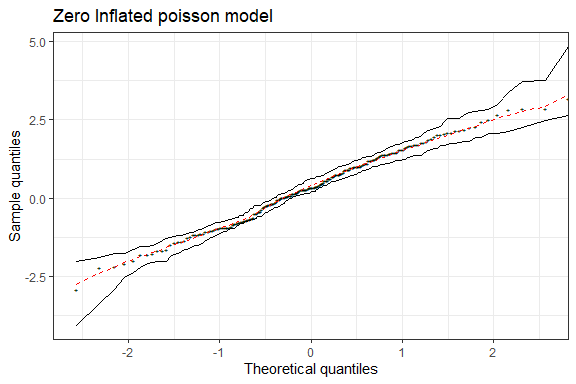All the sample RQR are within the envelopes.

Furthermore, the dispersion and the zero counts are now compatible with the assumed model.

plot(EPP_ZIP.gof,type="dispersion")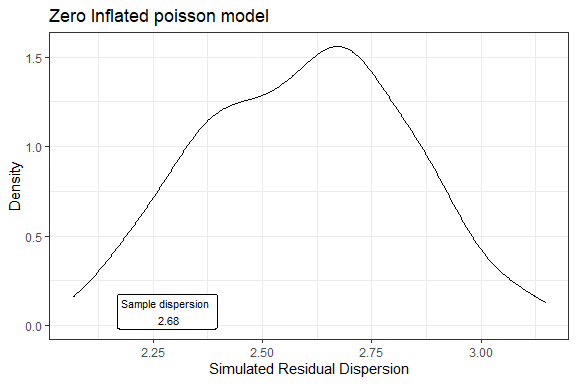DispersionTest(EPP_ZIP.gof)
#>         S   P_value
#>  2.675311 0.3960396
plot(EPP_ZIP.gof,type="zeros")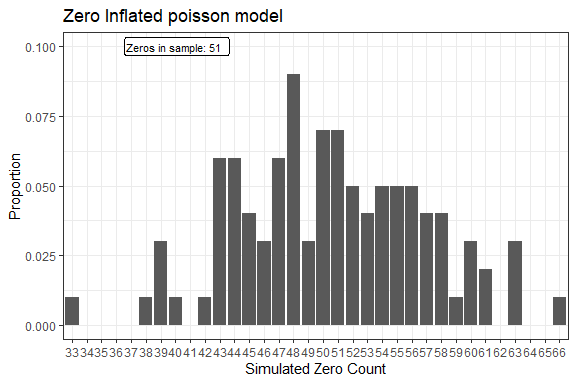ZeroTest(EPP_ZIP.gof)
#>  Count   P_value
#>     51 0.4950495